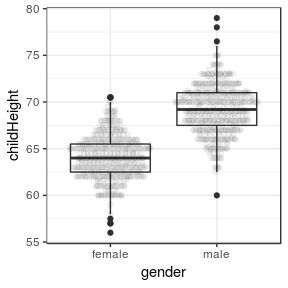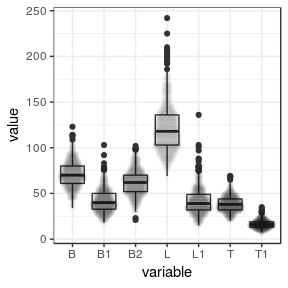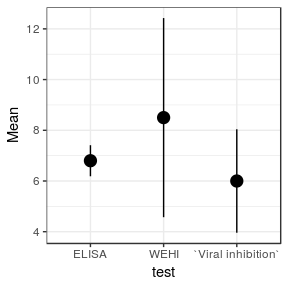Testing Coefficients of Variation from multiple samples

Preamble

If you use this package, please cite both the package and the paper that first presented the algorithm that you used (see below for details). Cite the package as follows:

Marwick, B. and K. Krishnamoorthy 2019 cvequality: Tests for the Equality of Coefficients of Variation from Multiple Groups. R software package version 0.1.3. Retrieved from https://github.com/benmarwick/cvequality, on 07/01/2019

And reference it in your text similar to this example:

“We used the R package cvequality (Version 0.1.3; Marwick and Krishnamoorthy 2019) to test for significant differences [etc.].”

A BibTeX entry for LaTeX users is:

@Manual{,
title = {cvequality: Tests for the Equality of Coefficients of Variation from Multiple Groups},
author = {Ben Marwick and Kalimuthu Krishnamoorthy},
note = {R package version 0.1.3},
url = {https://github.com/benmarwick/cvequality},
}

You can also access the citation details from the R console with citation(package = "cvequality")

Introduction

The coefficient of variation statistic is a simple and widely-used standardized measure of the spread of a set of measurements of a sample. It is a useful value because it allows us to directly compare variation in samples measured with different units, or with very different means. For example, with coefficients of variation we can compare the relative variability of lengths with that of weights, and so forth. It is also known as as relative standard deviation (RSD), and defined as the standard deviation divided by the mean:

$c_v = \frac{\sigma}{\mu}$

The coefficient of variation was introduced by Karl Pearson in 1896. Since then it has become widely used in chemistry, engineering, physics, sociology, finance, and other fields. Despite its ubiquity, we lack convenient methods for testing if $$c_v$$ values from different samples are equal or not. Numerous statistical methods have been described in publications, but until now, none have been implemented in an easy-to-use statistical package. This R package aims to remedy this situation by providing methods for testing for the equality of $$c_v$$ values from different samples. This will help researchers make decisions when comparing coefficients of variation.

Note that there are some limitations to using $$c_v$$ values. The main restriction is that $$c_v$$ should only be computed for data measured on a ratio scale, as these are the measurements that can only take non-negative values. For example it is not appropriate to compare $$c_v$$ of measurements made using Celsius and Fahrenheit, because these are interval scales that can take negative values and have arbitrary zero points. When the mean of a variable is zero, the $$c_v$$ cannot be computed, and when the mean is close to zero and the variable contains both positive and negative values, then the $$c_v$$ can be inaccurate. A lesser concern is that log-normal data have stationary $$c_v$$ values, and so they are generally uninformative.

What tests are included?

This package includes two tests:

• the ‘Asymptotic test for the equality of coefficients of variation from k populations’ (Feltz and Miller 1996)
• the ‘Modified signed-likelihood ratio test (SLRT) for equality of CVs’ (Krishnamoorthy and Lee 2014)

We include the Feltz and Miller (1996) test because it is widely cited as an authoritative test for the equality of $$c_v$$ values that performs better than many approximative tests. It is a ‘gold standard’ test for comparing multiple $$c_v$$. Our implementation is based on the following from Feltz and Miller (1996):

Let $$s_i$$ be the observed standard deviation of the $$i$$th sample (or group of measurements), $$x_i$$ be the observed mean of the $$i$$th sample, and let

$m_i=n_i-1$

and we do not know the population $$c_v$$, so let $$\widehat{\tau }_c$$ be an estimate as follows:

$\widehat{\tau }_c = \frac{\left( \sum _{j=1}^km_j\frac{S_j}{\bar{x}_j} \right)}{M}$

where

$M=\sum _{j=1}^m m_j$

Then the test statistic can be computed with:

\begin{aligned} D'A D= \widehat{\tau }_c^{-2}\left( 0.5+\widehat{\tau }_c^2\right) ^{-1}\left[ \sum _{i=1}^km_i\left(\frac{s_i}{\bar{x}_i} \right)^2-\frac{\widehat{\tau }_c^2}{M} \right] \end{aligned}

The $$D'AD$$ value measures how far each sample $$c_v$$ is from our estimate of the population $$c_v$$. Feltz and Miller (1996) note that the $$D'AD$$ value distributes as a central $$\chi^2$$ random variable with $$k-1$$ degrees of freedom, from which a p-value can be computed.

We include the Krishnamoorthy and Lee (2014) test as a more recent development with lower rates of type I error, better performance with uneven sample numbers, and more power across a range of conditions. The formulae for the Krishnamoorthy and Lee (2014) are quite involved, and we do not reproduce them here, but refer the reader to the paper.

How to use the tests?

For each test there are two functions, which we demonstrate below. The first function uses raw measurement data as its inputs. The second function uses only summary statistics as its inputs, intended for use when the raw data are not available.

To install the package:

install.packages("cvequality")

Using the functions with raw data of individual observations

To demonstrate the use the functions with raw data we will use Galton’s (1886) observations of heights (in inches) of 934 children in 205 families. This is one of the datasets that Pearson used when he introduced the $$c_v$$

Preview of first few rows of the GaltonFamilies data
X family father mother midparentHeight children childNum gender childHeight
1 001 78.5 67.0 75.43 4 1 male 73.2
2 001 78.5 67.0 75.43 4 2 female 69.2
3 001 78.5 67.0 75.43 4 3 female 69.0
4 001 78.5 67.0 75.43 4 4 female 69.0
5 002 75.5 66.5 73.66 4 1 male 73.5
6 002 75.5 66.5 73.66 4 2 male 72.5

Do male and female children have different variation in their heights? Let’s have a look, first with a plot to get some intuition about the variation:Their spreads look quite similar, let’s test our intuition, first with Feltz and Miller’s (1996) asymptotic test:

We can repeat the test with Krishnamoorthy and Lee’s (2014) modified signed-likelihood ratio test:

Summary of tests on the equality of CVs in child heights by gender
Test name Test statistic p-value
asymptotic 0.44164 0.5063320
M-SLRT 0.44164 0.5077081

We conclude that the $$c_v$$ values are very similar, and there is no difference in the variation of heights in male and female children.

For something a little different, let’s look at measurements of 600 stone artefacts from archaeological sites in Berkshire, England dating to the Lower Paleolithic (Fox and Baker 2006). We want to know if the length of the artefacts more variable than the other dimensions.

Preview of first few rows of the handaxes data
X Catalog L L1 B B1 B2 T T1
1 896.4.1 134 69 79 65 57 41 22
2 909.49.1 225 51 104 52 99 68 28
3 909.49.2 198 51 104 55 102 45 18
4 909.49.3 159 36 78 33 78 37 13
5 909.50.7 173 75 112 92 90 57 25
6 909.63.36 101 35 68 28 64 28 10

We need to reshape the data from the one-artefact-per-line format to a long format where the variable names are in one column, and the value for that variable is in another column:

Preview of first few rows of the handaxes data
variable value
L 134
L 225
L 198
L 159
L 173
L 101

Let’s have a look to get an idea of the variation in each variable:It looks like the L variable (length) has much greater variation that the others. Let’s test to see if this is due to chance or not:

Summary of tests on the equality of CVs in measurements of different variables of handaxe size
Test name Test statistic p-value
asymptotic 309.1919 0
M-SLRT 309.1919 0

We see low p-values (rounded to zero in the table the asymptotic test p-value is 8.76610110^{-64}), suggesting that the variation in $$c_v$$ values across different measurements is not due to chance. Length is more variable than the other dimensions of the handaxes.

Using the functions with summary statistics

In some circumstances we do not have access to the individual measurement data. For the situations we provide versions of the functions that take summary statistics as input.

Here’s an example using the same data that Feltz and Miller (1996) present in their paper. They provide mean, $$c_v$$, and n for three groups of measurements:

To test for the equality of these $$c_v$$ values, we need the standard deviations of the observations, which are easily obtained:

We can plot to see what to expect from the test:Looking at the plot, we might expect that the ELISA values have significantly less variation that the other two groups. Let’s see what the tests suggest:

The asymptotic test statistic is 5.5304524, which gives a p-value of 0.0629619. Assuming a typical value of 0.05 for $$\alpha$$, this test does not lead us to reject the hypothesis of no difference in variance. So we would conclude that the ELISA values are no less variable than the other two groups.

Let’s see how the modified signed-likelihood ratio test performs:

This results in for the test statistic, and a p-value of 0.036453. This p-value would lead us to reject the hypothesis of no difference in variance. So unlike the asymptotic test, this M-SLR test would conclude that the ELISA values are significantly less variable than the other two groups, consistent with our observations of the plot.

Obtaining reproducible results

We can use set.seed() before we run the function to ensure that we get the same output each time.

Here is some example data:

set.seed(42)
df <- data.frame(x = c(rnorm(20, 5, 2),
rnorm(20, 5, 4)),
y = c(rep(1, 20),
rep(2, 20)))

Here is the first run of the test, using set.seed()

set.seed(42)
mslr_test(nr = 10000, x = df$x, y = df$y)
## $MSLRT ##  5.827931 ## ##$p_value
##  0.01577366

And here is a second run of the same test, note that the set.seed() function must immediately preceed the statistic function:

set.seed(42)
mslr_test(nr = 10000, x = df$x, y = df$y)
## $MSLRT ##  5.827931 ## ##$p_value
##  0.01577366

Let’s scale it up to see if this is robust. We can re-run the same test 10 times with set.seed() before each run, and if the results are reproduible then we should expect to get the same output each time. We can test this by counting how many unique values are in the output of all the runs combined - it should be just one.

# repeat it n times to see how the results vary
n <- 10
reps <- replicate(n, {set.seed(42); mslr_test(nr = 10000, x = df$x, y = df$y)})

# take a look
stat <- unlist(reps[seq(1, length(reps), 2)])
pvals <- unlist(reps[seq(2, length(reps), 2)])

how_many_unique_values <- data.frame(unique_stat_values = length(unique(stat)),
unique_p_values = length(unique(pvals)))
kable(how_many_unique_values)
unique_stat_values unique_p_values
1 1